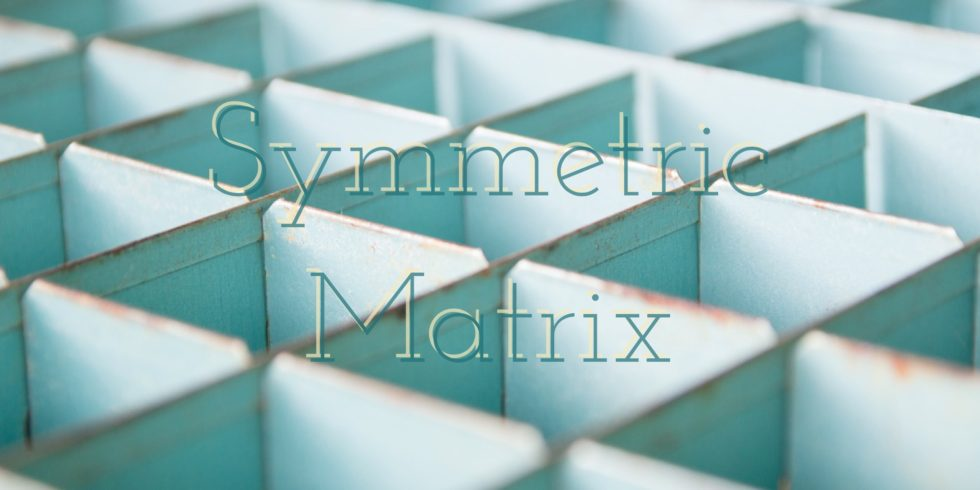# The set of $2\times 2$ Symmetric Matrices is a Subspace## Problem 586

Let $V$ be the vector space over $\R$ of all real $2\times 2$ matrices.
Let $W$ be the subset of $V$ consisting of all symmetric matrices.

(a) Prove that $W$ is a subspace of $V$.

(b) Find a basis of $W$.

(c) Determine the dimension of $W$.Add to solve later

## Proof.

Recall that $A$ is symmetric if $A^{\trans}=A$.

### (a) Prove that $W$ is a subspace of $V$.

We verify the following subspace criteria:

Subspace Criteria.

1. The zero vector of $V$ is in $W$.
2. For any $A, B\in W$, the sum $A+B\in W$.
3. For any $A\in W$ and $r\in \R$, the scalar product $rA\in W$.

The zero vector in $V$ is the $2\times 2$ zero matrix $O$.
It is clear that $O^{\trans}=O$, and hence $O$ is symmetric.
Thus $O\in W$ and condition 1 is met.

Let $A, B$ be arbitrary elements in $W$.
That is, $A$ and $B$ are symmetric matrices.
We show that the sum $A+B$ is also symmetric.
We have
\begin{align*}
(A+B)^{\trans}=A^{\trans}+B^{\trans}=A+B.
\end{align*}
The second equality follows as $A, B$ are symmetric.
Hence $A+B$ is symmetric and $A+B\in W$.
Condition 2 is met.

To check condition 3, let $A\in W$ and $r\in \R$.
We have
\begin{align*}
(rA)^{\trans}=rA^{\trans}=rA,
\end{align*}
where the second equality follows since $A$ is symmetric.
This implies that $rA$ is symmetric, and hence $rA\in W$.
So condition 3 is met, and we conclude that $W$ is a subspace of $V$ by subspace criteria.

### (b) Find a basis of $W$.

Let
$A=\begin{bmatrix} a_{11} & a_{12}\\ a_{21}& a_{22} \end{bmatrix}$ be an arbitrary element in the subspace $W$.
Then since $A^{\trans}=A$, we have
$\begin{bmatrix} a_{11} & a_{21}\\ a_{12}& a_{22} \end{bmatrix}=\begin{bmatrix} a_{11} & a_{12}\\ a_{21}& a_{22} \end{bmatrix}.$ This implies that $a_{12}=a_{21}$, and hence
\begin{align*}
A&=\begin{bmatrix}
a_{11} & a_{12}\\
a_{12}& a_{22}
\end{bmatrix}\6pt] &=a_{11}\begin{bmatrix} 1 & 0\\ 0& 0 \end{bmatrix}+a_{12}\begin{bmatrix} 0 & 1\\ 1& 0 \end{bmatrix}+a_{22}\begin{bmatrix} 0 & 0\\ 0& 1 \end{bmatrix}. \end{align*} Let B=\{v_1, v_2, v_3\}, where v_1, v_2, v_3 are 2\times 2 matrices appearing in the above linear combination of A. Note that these matrices are symmetric. Hence we showed that any element in W is a linear combination of matrices in B. Thus B is a spanning set for the subspace W. We show that B is linearly independent. Suppose that we have \[c_1v_1+c_2v_2+c_3v_3=\begin{bmatrix} 0 & 0\\ 0& 0 \end{bmatrix}. Then it follows that
$\begin{bmatrix} c_1 & c_2\\ c_2& c_3 \end{bmatrix}=\begin{bmatrix} 0 & 0\\ 0& 0 \end{bmatrix}.$ Thus $c_1=c_2=c_3=$ and the set $B$ is linearly independent.

As $B$ is a linearly independent spanning set, we conclude that $B$ is a basis for the subspace $W$.

### (c) Determine the dimension of $W$.

Recall that the dimension of a subspace is the number of vectors in a basis of the subspace.

In part (b), we found that $B=\{v_1, v_2, v_3\}$ is a basis for the subspace $W$.
As $B$ consists of three vectors, the dimension of $W$ is $3$.

## Related Question (Skew-Symmetric Matrices)

A matrix $A$ is called skew-symmetric if $A^{\trans}=-A$.

Problem.
Let $V$ be the vector space of all $2\times 2$ matrices.
Let $W$ be a subset of $V$ consisting of all $2\times 2$ skew-symmetric matrices.

Prove that $W$ is a subspace of $V$ and also find a basis and dimension of $W$.

The solution is given in the post ↴
Subspace of Skew-Symmetric Matrices and Its DimensionAdd to solve later

### 1 Response

1. 10/17/2017

[…] The solution is given in the post ↴ The set of $2times 2$ Symmetric Matrices is a Subspace […]

This site uses Akismet to reduce spam. Learn how your comment data is processed.

###### More in Linear Algebra##### Diagonalize the $2\times 2$ Hermitian Matrix by a Unitary Matrix

Consider the Hermitian matrix $A=\begin{bmatrix} 1 & i\\ -i& 1 \end{bmatrix}.$ (a) Find the eigenvalues of $A$. (b) For each...

Close